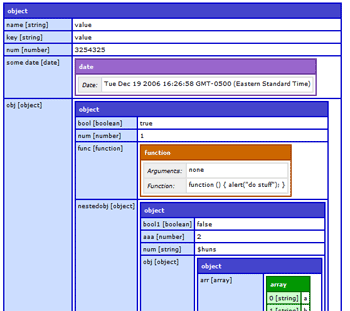## 如何列出 JavaScript 对象的属性？

``````var myObject =
{"ircEvent": "PRIVMSG", "method": "newURI", "regex": "^http://.*"};``````
``keys == ["ircEvent", "method", "regex"]``

## 答案

``var keys = Object.keys(myObject);``

``````var getKeys = function(obj){
var keys = [];
for(var key in obj){
keys.push(key);
}
return keys;
}``````

``````for (var key in obj) {
if (obj.hasOwnProperty(key)) {
/* useful code here */
}
}``````

``````Object.keys = Object.keys || (function () {
var hasOwnProperty = Object.prototype.hasOwnProperty,
hasDontEnumBug = !{toString:null}.propertyIsEnumerable("toString"),
DontEnums = [
'toString', 'toLocaleString', 'valueOf', 'hasOwnProperty',
'isPrototypeOf', 'propertyIsEnumerable', 'constructor'
],
DontEnumsLength = DontEnums.length;

return function (o) {
if (typeof o != "object" && typeof o != "function" || o === null)
throw new TypeError("Object.keys called on a non-object");

var result = [];
for (var name in o) {
if (hasOwnProperty.call(o, name))
result.push(name);
}

if (hasDontEnumBug) {
for (var i = 0; i < DontEnumsLength; i++) {
if (hasOwnProperty.call(o, DontEnums[i]))
result.push(DontEnums[i]);
}
}

return result;
};
})();``````

``````var o = {"foo": 1, "bar": 2};

ECMAScript 5 兼容性表： http : //kangax.github.com/es5-compat-table/

`Object.getOwnPropertyNames(obj)`

``````var o = Object.create({base:0})
Object.defineProperty(o, 'yes', {enumerable: true})
Object.defineProperty(o, 'not', {enumerable: false})

console.log(Object.getOwnPropertyNames(o))
// [ 'yes', 'not' ]

console.log(Object.keys(o))
// [ 'yes' ]

for (var x in o)
console.log(x)
// yes, base``````

• `Object.getOwnPropertyNames``Object.keys` 不会沿着原型链向上寻找`base`
• `for in`

http://ajaxian.com/archives/javascript-variable-dump-in-coldfusion``````var objectKeys = \$.map(object, function(value, key) {
return key;
});``````

``````this.getKeys = function() {

var keys = new Array();
for(var key in this) {

if( typeof this[key] !== 'function') {

keys.push(key);
}
}
return keys;
}``````

``````var myJSONObject =  {"ircEvent": "PRIVMSG", "method": "newURI", "regex": "^http://.*"};
var keys=[];
for (var i in myJSONObject ) { keys.push(i); }
``[i for(i in obj)]``